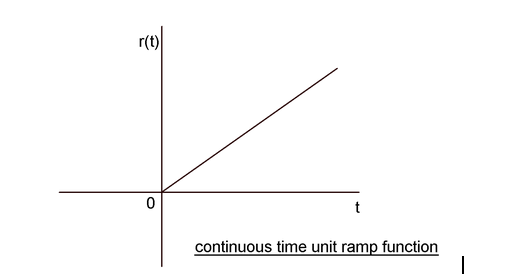# Definition of unit impulse function

For a Unit ramp function area of pulse curve is unity

Options:

a) discontinuous at time t=0
b) starts at time t=0 and linearly increases with t
c) none of the above

Correct Answer: b) starts at time t=0 and linearly increases with t

Explanation:A Unit ramp function is a continuous time function that starts at time t=0 and linearly increases with t. Denoted by r (t), the unit ramp function is represented by
r(t) = 0 for t<0
= t for t>0